1595572800

# Every DataFrame Manipulation, Explained & Visualized Intuitively

Pandas offers a wide range of DataFrame manipulations, but many of them are complex and may not seem approachable. This article will present 8 essential DataFrame manipulation methods which cover almost all of the manipulation functions a data scientist would need to know. Each method will include an explanation, visualization, code, and tricks to remember it.

## Pivot

Pivoting a table creates a new ‘pivoted table’ that projects existing columns in the data as elements of a new table, being the index, column, and the values. The columns in the initial DataFrame that will become the index and the columns are displayed as unique values, and combinations of these two columns will be displayed as the value. This means that pivots cannot handle duplicate values.

The code to pivot a DataFrame named `df` is as follows:

``````df.pivot(index='foo', columns='bar', values='baz')
``````

To memorize: A pivot is — outside the realm of data manipulation — a turn around some sort of object. In sports, one can ‘pivot’ around their foot to spin: pivots in pandas are similar. The state of the original DataFrame are pivoted around central elements of a DataFrame into a new one. Some elements very literally pivot in that they are rotated or transformed(like column ‘`bar`’).

## Melt

Melting can be thought of as an ‘unpivot’, in that it converts a matrix-based data (has two dimensions) into list-based data (columns represent values and rows indicate unique data points), whereas pivots do the opposite. Consider a two-dimensional matrix with one dimension ‘`B`’ and ‘`C`’ (column names), with the other dimension ‘`a`’, ‘`b`’, and ‘`c`’ (row indices).

We select an ID, one of the dimensions, and a column/columns to contain values. The column(s) that contain values are transformed into two columns: one for the variable (the name of the value column) and another for the value (the number contained in it).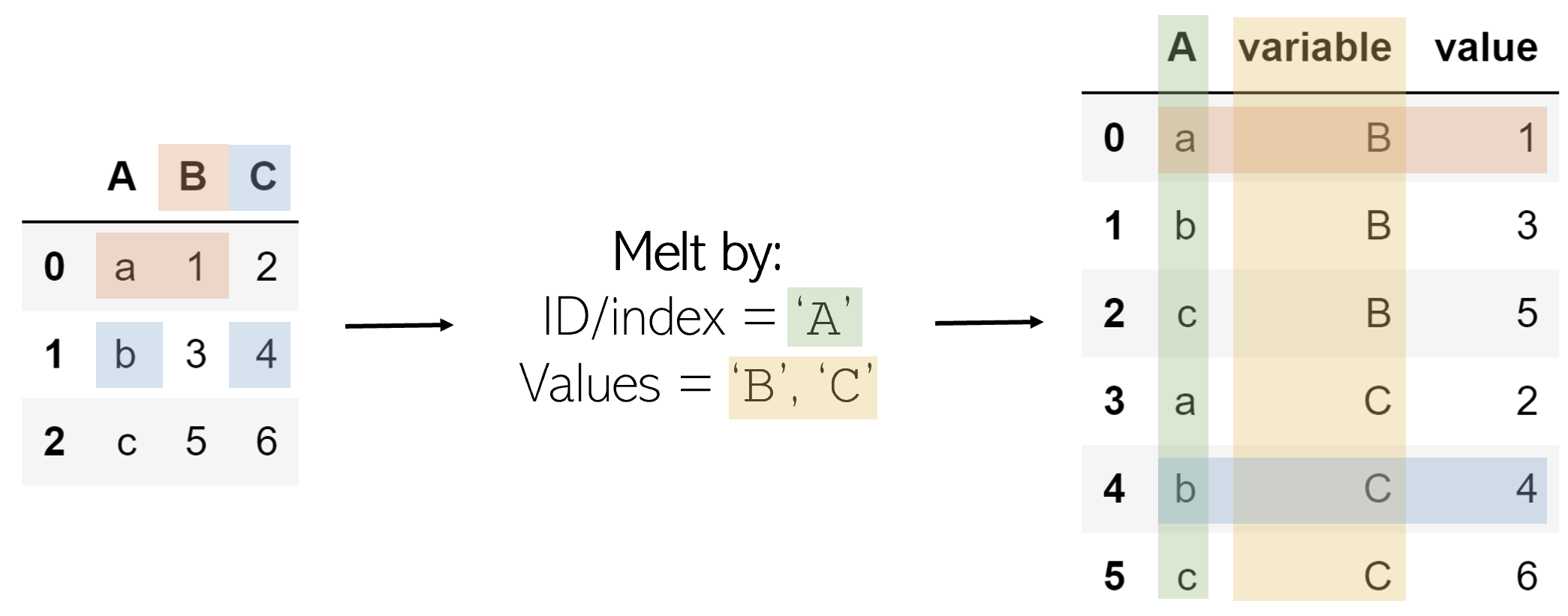The result is every combination of the ID column’s values (`a``b``c`) and the value columns (`B``C`), with its corresponding value, organized in list format.

The melt operation can be performed like such on DataFrame `df`:

``````df.melt(id_vars=['A'], value_vars=['B','C'])
``````

To memorize: Melting something like a candle is to turn a solidified and composite object into several much smaller, individual elements (wax droplets). Melting a two-dimensional DataFrame unpacks its solidified structure and records its pieces as individual entries in a list.

## Explode

Exploding is a helpful method to get rid of lists in the data. When a column is exploded, all lists inside of it are listed as new rows under the same index (to prevent this, simply call `.reset_index()` afterwards). Non-list items like strings or numbers are not affected, and empty lists are NaN values (you can cleanse these using `.dropna()`).

#computer-science #data-science #data #data-analysis #programming #data analysis

## Buddha Community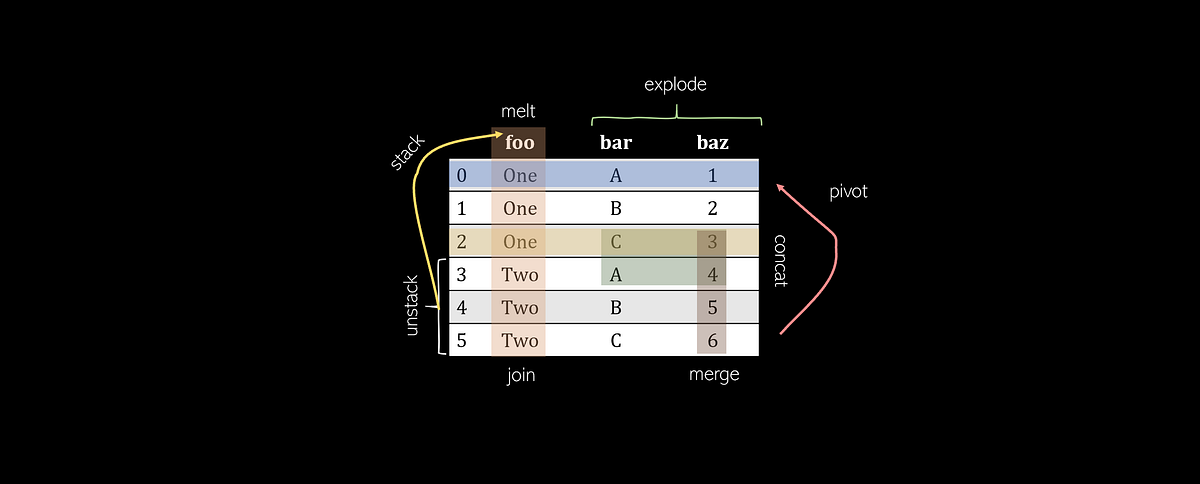1591177440

## Visual Analytics and Advanced Data Visualization

Visual Analytics is the scientific visualization to emerge an idea to present data in such a way so that it could be easily determined by anyone.

It gives an idea to the human mind to directly interact with interactive visuals which could help in making decisions easy and fast.

Visual Analytics basically breaks the complex data in a simple way.

The human brain is fast and is built to process things faster. So Data visualization provides its way to make things easy for students, researchers, mathematicians, scientists e

#blogs #data visualization #business analytics #data visualization techniques #visual analytics #visualizing ml models1623927960

## Pandas DataFrame vs. Spark DataFrame: When Parallel Computing Matters

### With Performance Comparison Analysis and Guided Example of Animated 3D Wireframe Plot

Python is famous for its vast selection of libraries and resources from the open-source community. As a Data Analyst/Engineer/Scientist, one might be familiar with popular packages such as NumpyPandasScikit-learnKeras, and TensorFlow. Together these modules help us extract value out of data and propels the field of analytics. As data continue to become larger and more complex, one other element to consider is a framework dedicated to processing Big Data, such as Apache Spark. In this article, I will demonstrate the capabilities of distributed/cluster computing and present a comparison between the Pandas DataFrame and Spark DataFrame. My hope is to provide more conviction on choosing the right implementation.

### Pandas DataFrame

Pandas has become very popular for its ease of use. It utilizes DataFrames to present data in tabular format like a spreadsheet with rows and columns. Importantly, it has very intuitive methods to perform common analytical tasks and a relatively flat learning curve. It loads all of the data into memory on a single machine (one node) for rapid execution. While the Pandas DataFrame has proven to be tremendously powerful in manipulating data, it does have its limits. With data growing at an exponentially rate, complex data processing becomes expensive to handle and causes performance degradation. These operations require parallelization and distributed computing, which the Pandas DataFrame does not support.

### Introducing Cluster/Distribution Computing and Spark DataFrame

Apache Spark is an open-source cluster computing framework. With cluster computing, data processing is distributed and performed in parallel by multiple nodes. This is recognized as the MapReduce framework because the division of labor can usually be characterized by sets of the mapshuffle, and reduce operations found in functional programming. Spark’s implementation of cluster computing is unique because processes 1) are executed in-memory and 2) build up a query plan which does not execute until necessary (known as lazy execution). Although Spark’s cluster computing framework has a broad range of utility, we only look at the Spark DataFrame for the purpose of this article. Similar to those found in Pandas, the Spark DataFrame has intuitive APIs, making it easy to implement.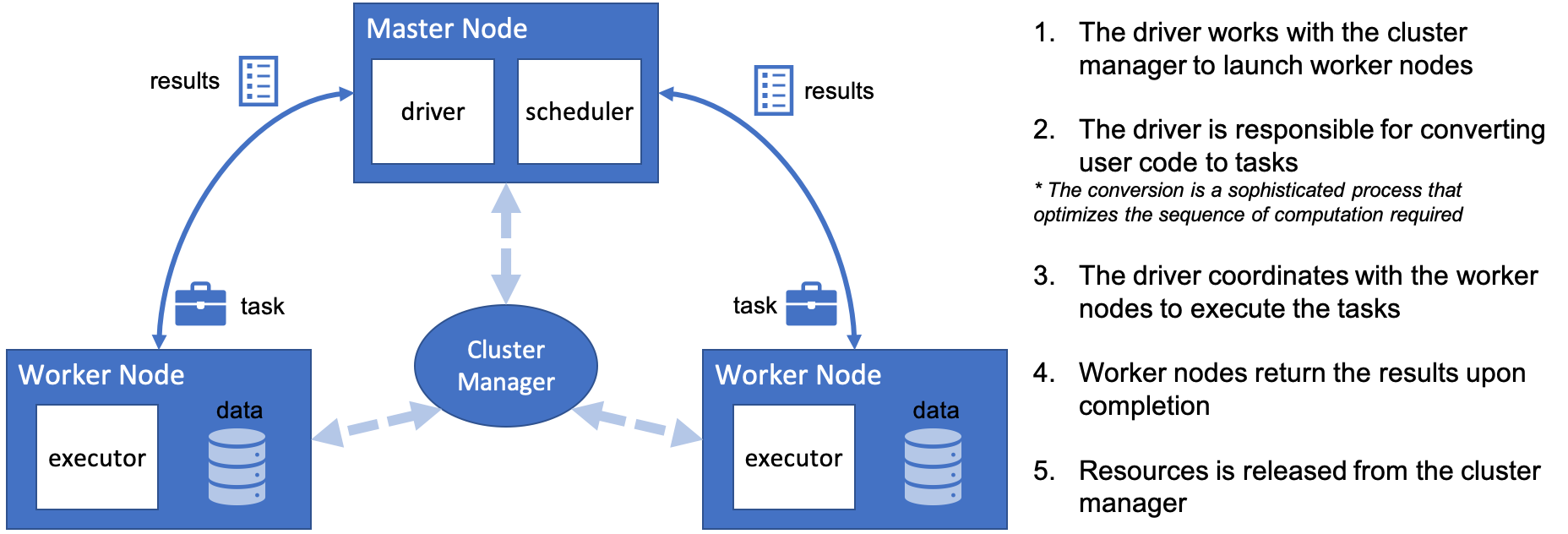#pandas dataframe vs. spark dataframe: when parallel computing matters #pandas #pandas dataframe #pandas dataframe vs. spark dataframe #spark #when parallel computing matters1596780780

## Every DataFrame Manipulation, Explained & Visualized Intuitively

Pandas offers a wide range of DataFrame manipulations, but many of them are complex and may not seem approachable. This article will present 8 essential DataFrame manipulation methods which cover almost all of the manipulation functions a data scientist would need to know. Each method will include an explanation, visualization, code, and tricks to remember it.

## Pivot

Pivoting a table creates a new ‘pivoted table’ that projects existing columns in the data as elements of a new table, being the index, column, and the values. The columns in the initial DataFrame that will become the index and the columns are displayed as unique values, and combinations of these two columns will be displayed as the value. This means that pivots cannot handle duplicate values.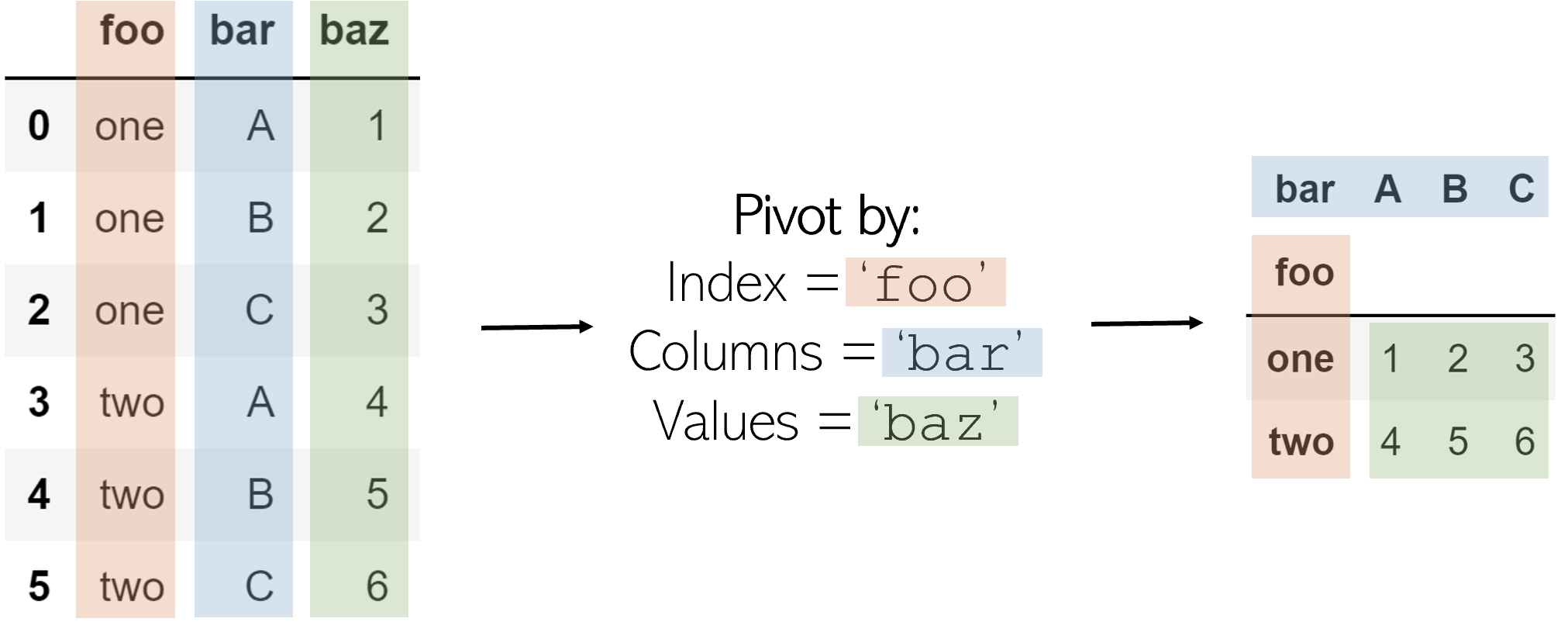The code to pivot a DataFrame named `df` is as follows:

``````df.pivot(index='foo', columns='bar', values='baz')
``````

To memorize: A pivot is — outside the realm of data manipulation — a turn around some sort of object. In sports, one can ‘pivot’ around their foot to spin: pivots in pandas are similar. The state of the original DataFrame are pivoted around central elements of a DataFrame into a new one. Some elements very literally pivot in that they are rotated or transformed(like column ‘`bar`’).

## Melt

Melting can be thought of as an ‘unpivot’, in that it converts a matrix-based data (has two dimensions) into list-based data (columns represent values and rows indicate unique data points), whereas pivots do the opposite. Consider a two-dimensional matrix with one dimension ‘`B`’ and ‘`C`’ (column names), with the other dimension ‘`a`’, ‘`b`’, and ‘`c`’ (row indices).

We select an ID, one of the dimensions, and a column/columns to contain values. The column(s) that contain values are transformed into two columns: one for the variable (the name of the value column) and another for the value (the number contained in it).The result is every combination of the ID column’s values (`a``b``c`) and the value columns (`B``C`), with its corresponding value, organized in list format.

The melt operation can be performed like such on DataFrame `df`:

``````df.melt(id_vars=['A'], value_vars=['B','C'])
``````

To memorize: Melting something like a candle is to turn a solidified and composite object into several much smaller, individual elements (wax droplets). Melting a two-dimensional DataFrame unpacks its solidified structure and records its pieces as individual entries in a list.

## Explode

Exploding is a helpful method to get rid of lists in the data. When a column is exploded, all lists inside of it are listed as new rows under the same index (to prevent this, simply call `.reset_index()` afterwards). Non-list items like strings or numbers are not affected, and empty lists are NaN values (you can cleanse these using `.dropna()`).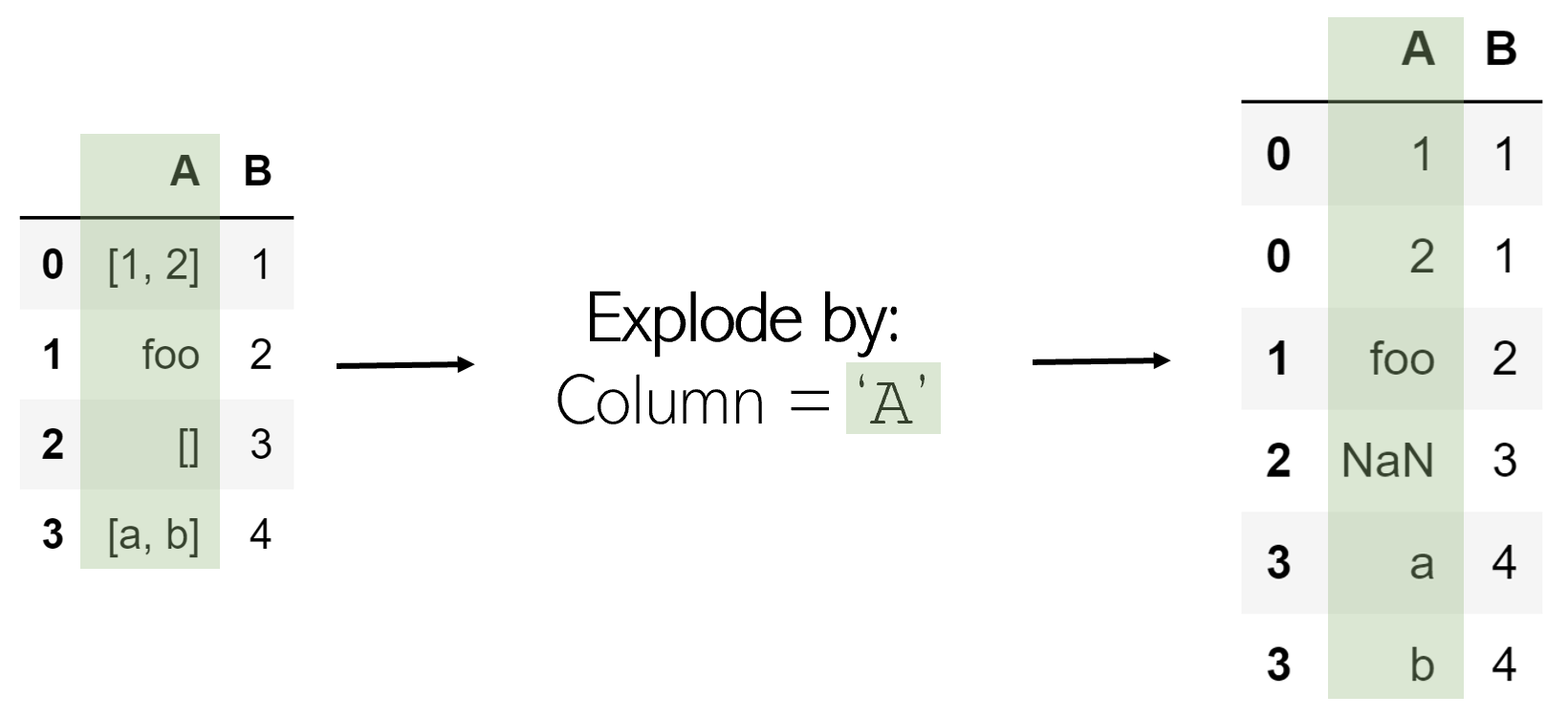Exploding a column `‘A’` in DataFrame `df` is very simple:

``````df.explode(‘A’)
``````

To remember: Exploding something releases all its internal contents — exploding a list separates its elements.

#computer-science #data-science #data #data-analysis #programming #data analysis1595572800

## Every DataFrame Manipulation, Explained & Visualized Intuitively

Pandas offers a wide range of DataFrame manipulations, but many of them are complex and may not seem approachable. This article will present 8 essential DataFrame manipulation methods which cover almost all of the manipulation functions a data scientist would need to know. Each method will include an explanation, visualization, code, and tricks to remember it.

## Pivot

Pivoting a table creates a new ‘pivoted table’ that projects existing columns in the data as elements of a new table, being the index, column, and the values. The columns in the initial DataFrame that will become the index and the columns are displayed as unique values, and combinations of these two columns will be displayed as the value. This means that pivots cannot handle duplicate values.

The code to pivot a DataFrame named `df` is as follows:

``````df.pivot(index='foo', columns='bar', values='baz')
``````

To memorize: A pivot is — outside the realm of data manipulation — a turn around some sort of object. In sports, one can ‘pivot’ around their foot to spin: pivots in pandas are similar. The state of the original DataFrame are pivoted around central elements of a DataFrame into a new one. Some elements very literally pivot in that they are rotated or transformed(like column ‘`bar`’).

## Melt

Melting can be thought of as an ‘unpivot’, in that it converts a matrix-based data (has two dimensions) into list-based data (columns represent values and rows indicate unique data points), whereas pivots do the opposite. Consider a two-dimensional matrix with one dimension ‘`B`’ and ‘`C`’ (column names), with the other dimension ‘`a`’, ‘`b`’, and ‘`c`’ (row indices).

We select an ID, one of the dimensions, and a column/columns to contain values. The column(s) that contain values are transformed into two columns: one for the variable (the name of the value column) and another for the value (the number contained in it).The result is every combination of the ID column’s values (`a``b``c`) and the value columns (`B``C`), with its corresponding value, organized in list format.

The melt operation can be performed like such on DataFrame `df`:

``````df.melt(id_vars=['A'], value_vars=['B','C'])
``````

To memorize: Melting something like a candle is to turn a solidified and composite object into several much smaller, individual elements (wax droplets). Melting a two-dimensional DataFrame unpacks its solidified structure and records its pieces as individual entries in a list.

## Explode

Exploding is a helpful method to get rid of lists in the data. When a column is exploded, all lists inside of it are listed as new rows under the same index (to prevent this, simply call `.reset_index()` afterwards). Non-list items like strings or numbers are not affected, and empty lists are NaN values (you can cleanse these using `.dropna()`).

#computer-science #data-science #data #data-analysis #programming #data analysis1591184760

## Visual Analytics Services for Data-Driven Decision Making

Visual analytics is the process of collecting, examining complex and large data sets (structured or unstructured) to get useful information to draw conclusions about the datasets and visualize the data or information in the form of interactive visual interfaces and graphical manner.

Data analytics is usually accomplished by extracting or collecting data from different data sources in the form of numbers, statistics and overall activity of any organization, with different deep learning and analytics tools, which is then processed using data visualization software and presented in the form of graphical charts, figures, and bars.

In today technology world, data are reproduced in incredible rate and amount. Visual Analytics helps the world to make the vast and complex amount of data useful and readable. Visual Analytics is the process to collect and store the data at a faster rate than analyze the data and make it helpful.

As human brain process visual content better than it processes plain text. So using advanced visual interfaces, humans may directly interact with the data analysis capabilities of today’s computers and allow them to make well-informed decisions in complex situations.

It allows you to create beautiful, interactive dashboards or reports that are immediately available on the web or a mobile device. The tool has a Data Explorer that makes it easy for the novice analyst to create forecasts, decision trees, or other fancy statistical methods.

#blogs #data visualization #data visualization tools #visual analytics #visualizing ml models